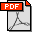P. K. Agarwal, M. de Berg, J. Gao, L. J. Guibas, and S. Har-Peled, Staying in the Middle: Exact and Approximate Medians in R1 and R2 for Moving Points, Proc. of the 17-th Canadian Conference on Computational Geometry (CCCG’05), 42-45, August, 2005. Abstract: Many divide-and-conquer based geometric algorithms and order-statistics problems ask for a point that lies “in the middle” of a given point set. We study several fundamental problems of this type for moving points in one and two dimensions. In particular, we show how to kinetically maintain the median of a set of n points moving on the real line, and a center point of a set of n points moving in the plane, that is, a point such that any line through it has at most 2n/3 on either side of it. Since the maintenance of exact medians and center points can be quite expensive, we also show how to maintain epsilon-approximate medians and center points and argue that the latter can be made to be much more stable under motion. These results are based on a new algorithm to maintain an epsilon-approximation of a range space under insertions and deletions, which is of independent interest. All our approximation algorithms run in near-linear time. Bibtex: ```@inproceedings{abggh-smeamRRmp-05, author="Pankaj K. Agarwal, Mark de Berg, Jie Gao, Leonidas J. Guibas, Sariel Har-Peled", title="Staying in the Middle: Exact and Approximate Medians in \$R^1\$ and \$R^2\$ for Moving Points", booktitle="Proc. of the 17th Canadian Conference on Computational Geometry (CCCG・5)", pages="42--45", month="August", year="2005" } ```